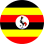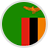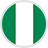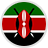Draw Time
Every 15 Minutes
DIRECT
5 numbers are drawn during draw.
If your selected number/s is part of the first 5 winning numbers drawn then you are considered as a winner.
Direct 2: Pick two numbers from the 90 numbers provided; your numbers can be among the five lucky numbers selected in the draw.
Direct 3: Pick three numbers from the 90 numbers provided; your numbers can be among the five lucky numbers selected in the draw
PERM2
With Perm 2, you begin by picking multiple random numbers - a minimum of 3 numbers and a maximum of 22 numbers.
As you pick your random numbers, you will be able to view the number of lines these selected numbers can be combined to form in sets of two. The system calculates these permutations based on a globally accepted mathematical formula.
The result of winning numbers is published within every 15-minute interval. The result is by standard a line of five numbers.
You win when any of your lines of two (formed from your randomly selected numbers) can be found within the published result.
Payout Calculation: Number of Lines Matched x Stake Per Line x Odds
Example:
If player A selected 3 numbers, such as 14, 26 and 58; only three lines of possible combinations will be generated as following:
Line 1: 14,26
Line 2: 14,58
Line 3: 26,58
Since stake per line is ₦10, Total stake will be ₦30.
Assuming the winning numbers (i.e. the announced result) are 3,9,26,39,58; only Line 3 from your selected numbers combination will match because that exact line appears in the winning numbers sequence.
Since only one of your three lines can be found in the winning numbers, your payout shall be calculated thus:  1(matched line number) x ₦10(stake per line) x 300(odds)=₦₦9,000.
This game might sound complicated, but once you register for your account and you discover several  ways of betting, you will love it.
PERM3
With Perm 3, you begin by picking multiple random numbers - a minimum of 4 numbers and a maximum of 22 numbers.
As you pick your random numbers, you will be able to view the number of lines these selected numbers can be combined to form in sets of three. The system calculates these permutations based on a globally accepted mathematical formula.
The result of winning numbers is published within every 15-minute interval. The result is by standard a line of five numbers.
You win when any of your lines of three (formed from your randomly selected numbers) can be found within the published result.
Payout Calculation: Number of Lines Matched x Stake Per Line x Odds
Example:
If player A selected 4 numbers, such as 11, 24, 39 and 65; only four lines of possible combinations will be generated as following:
Line 1: 11,24,39
Line 2: 11,24,65
Line 3: 11,39,65
Line 4: 24,39,65
Since stake per line is ₦10, Total stake will be ₦40.
Assuming the winning numbers (i.e. the announced result) are 5,6,24,39,65; only Line 4 from your selected numbers combination will match because that exact line appears in the winning numbers sequence.
Since only one of your four lines can be found in the winning numbers, your payout shall be calculated thus:  1(matched line number) x ₦10(stake per line) x 2,500(odds)=₦25,000.
This game might sound complicated, but once you register for your account and you discover several  ways of betting, you will love it.
BET TYPE PRIZE LEVEL ODDS
Direct 5 5/5 48,000
Direct 4 4/5 6,600
Direct 3 3/5 2,500
Direct 2 2/5 300
Perm 3 3/5 2,500
Perm 2 2/5 300UgandaZambiaNigeriaKenya